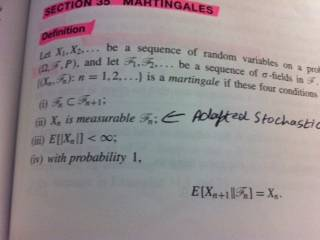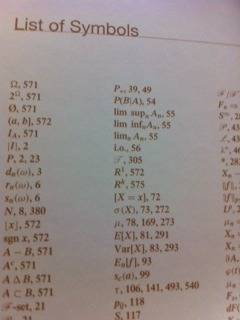# What is E[|X|] ?

woundedtiger4
Hi all,
in probability what does E[|X|] mean? If I am not wrong |X| means absolute but when we use |...| (perpendicular bars) then what does it mean when we use it in sense of probability?

Last edited:

|X| is the absolute value of X, i.e. √X^2.

woundedtiger4
|X| is the absolute value of X, i.e. √X^2.

so when we are saying that E[|X|] then we are taking an absolute value of X ?

woundedtiger4
Also, in martingale when we use E[X_n+1 || F_n] = X_n then the two perpendicular bars || denotes conditional probability or what?

so when we are saying that E[|X|] then we are taking an absolute value of X ?

Yes, it is the expectation value of the absolute value of x. E.g. if X has a uniform distribution in -1<=x<-1 then E(X)=0 but |X| has a uniform distribution 0<=|x|<=1, so that E(|X|)=0.5.
No idea about the double bar in Martingales. Usually, this nomenclature is explained at the beginning of the article or book where it is used.

woundedtiger4
Yes, it is the expectation value of the absolute value of x. E.g. if X has a uniform distribution in -1<=x<-1 then E(X)=0 but |X| has a uniform distribution 0<=|x|<=1, so that E(|X|)=0.5.
No idea about the double bar in Martingales. Usually, this nomenclature is explained at the beginning of the article or book where it is used.

thank you sir

unfortunately in my book it is not explained :(

Homework Helper
I simply don't believe that a book on probability would use "E(x)" without explaining that it means the expected value, and explaining with at expected value meant. I can believe that it would not define the absolute value because you should have learned that in a basic algebra class. And your question, as stated, doesn't really have anything to do with "martingales".

woundedtiger4
I simply don't believe that a book on probability would use "E(x)" without explaining that it means the expected value, and explaining with at expected value meant. I can believe that it would not define the absolute value because you should have learned that in a basic algebra class. And your question, as stated, doesn't really have anything to do with "martingales".

Yes, you won't believe that a book on probability would use "E(x)" without explaining that it means the expected value because you didn't even bother to read the whole thread or at least my last message in which I have quoted the message of gentleman "DrDu" in which he has clearly told me to look at the beginning of the book where the list of symbols is given, and then I replied that unfortunately my book doesn't give sufficient information.

I know what is expected value but I don't know the meaning of "||" in martingale, does this symbol refers to conditional (expectation/probability).in the book it tells about first 3 conditions but unfortunately the 4th condition, in which the symbol double bar ("||") has been used, has not been described very well or perhaps I don't understand it.in this image you can see that the list of symbol has been given in the beginning of the book but unfortunately it doesn't contain "||" and this list only tells (by giving the corresponding page numbers) that where these symbols have been used (for the first time) in this book.

I also suppose that || means conditional expectation in that case, especially as it coincides with the definition in Wikipedia. However if you have doubt you have to look through the book where this nomenclature first appears. I did not say that it necessarily appears in the list of symbols. Most books lack a list of symbols at all.

woundedtiger4
I simply don't believe that a book on probability would use "E(x)" without explaining that it means the expected value, and explaining with at expected value meant. I can believe that it would not define the absolute value because you should have learned that in a basic algebra class. And your question, as stated, doesn't really have anything to do with "martingales".

I also suppose that || means conditional expectation in that case, especially as it coincides with the definition in Wikipedia. However if you have doubt you have to look through the book where this nomenclature first appears. I did not say that it necessarily appears in the list of symbols. Most books lack a list of symbols at all.

Thanks

devinedj
in the book it tells about first 3 conditions but unfortunately the 4th condition, in which the symbol double bar ("||") has been used, has not been described very well or perhaps I don't understand it.

I don't have much knowledge of Martingales but the 4th condition guarantees that the distribution of each subsequent random variable, X_{n+1} is centered around X_n in F_n.

As an example, let our random variable X_n represent the position after n steps in a one-dimensional random walk. The fourth condition imposes that it is equally likely to step forward (to X_n + 1) as it is to step backwards (to X_n - 1). It's really just a conditional probability problem since the position after n+1 steps depends on the position after n steps.